# A Quick Introduction to iNextPD via Examples

#### 2017-03-11

`iNextPD` (iNterpolation and extrapolation for Phylogenetic Diversity) is an R package provides the rarefaction and extrapolation framework to making fair comparison of abundance-sensitive phylogenetic diversity among multiple assemblages (Hsieh and Chao, Systematic Biology, 2016). In this document, we provide a quick introduction demonstrating how to run `iNextPD`. Detailed information about `iNextPD` functions is provided in the iNextPD Manual, also available in CRAN. See Chao et al. (2015) and Hsieh and Chao (2016) for methodologies. An online version of `PhDOnline` (https://chao.shinyapps.io/PhDOnline/) is also available for users without an R background. A neutral theory of species diversity is included in Chao et al. (2014); and a brief description of methods and R package (`iNEXT`) are included in an application paper by Hsieh, Ma & Chao (2016).

`iNextPD` is an extension for `iNEXT`, which extending trdional rarefaction and extrapoltion framework for species diversity to abundance-sensitive phylogenetic diversity. `iNextPD` focuses on three measures of Hill numbers of order q: Faith’s PD (`q = 0`), a simple transformation of phylogenetic entropy (`q = 1`) and and a simple transformation of Rao’s quadratic entropy (`q = 2`). For each diversity measure, `iNextPD` uses the observed sample of abundance or incidence data (called the “reference sample”) to compute diversity estimates and the associated 95% confidence intervals for the following two types of rarefaction and extrapolation (R/E):

1. Sample‐size‐based R/E sampling curves: `iNextPD` computes diversity estimates for rarefied and extrapolated samples up to an appropriate size. This type of sampling curve plots the diversity estimates with respect to sample size (`tyep=1`).
2. Coverage‐based R/E sampling curves: `iNextPD` computes diversity estimates for rarefied and extrapolated samples with sample completeness (as measured by sample coverage) up to an appropriate coverage. This type of sampling curve plots the diversity estimates with respect to sample coverage (`type=3`).

`iNextPD` also plots the above two types of sampling curves and a sample completeness curve. The sample completeness curve provides a bridge between these two types of curves (`type=2`).

## HOW TO RUN INEXTPD:

The `iNextPD` package is available on CRAN and can be downloaded with a standard R installation procedure using the following commands. For a first‐time installation, the additional visualization extension packages (`ade4`, `ggplot2`, `iNEXT`, `Rcpp`) must be loaded.

``````## install iNEXT package from CRAN
install.packages("iNextPD")

install.packages('devtools')
library(devtools)
install_github('JohnsonHsieh/iNextPD')

## import packages
library(iNextPD)
library(ggplot2)

Remark: In order to install `devtools` package, you should update R to the latest version. Also, to get `install_github` to work, you should install the `httr` package.

## MAIN FUNCTION: `iNextPD()`

We first describe the main function `iNextPD()` with default arguments:

``iNextPD(x, labels, phy, q=0, datatype="abundance", size=NULL, endpoint=NULL, knots=40, se=FALSE, conf=0.95, nboot=50)``
The arguments of this function are briefly described below, and will be explained in more details by illustrative examples in later text. This main function computes diversity estimates of order q = 0, 1, 2, the sample coverage estimates and related statistics for K (if `knots=K`) evenly‐spaced knots (sample sizes) between size 1 and the `endpoint`, where the endpoint is described below. Each knot represents a particular sample size for which diversity estimates will be calculated. By default, endpoint = double the reference sample size (total sample size for abundance data; total sampling units for incidence data). For example, if `endpoint = 10`, `knot = 4`, diversity estimates will be computed for a sequence of samples with sizes (1, 4, 7, 10).
Argument Description
`x` a `matrix`, `data.frame`, `lists` of species abundances or incidence data.
`labels` species names for object `x`.
`phy` a `phylog` objcet for input phylo-tree.
`q` a number or vector specifying the diversity order(s) of Hill numbers.
`datatype` data type of input data: individual-based abundance data (`datatype = "abundance"`), or species by sampling-units incidence matrix (`datatype = "incidence_raw"`).
`size` an integer vector of sample sizes for which diversity estimates will be computed. If `NULL`, then diversity estimates will be calculated for those sample sizes determined by the specified/default `endpoint` and `knots`.
`endpoint` an integer specifying the sample size that is the endpoint for R/E calculation; If `NULL`, then `endpoint=`double the reference sample size.
`knots` an integer specifying the number of equally-spaced `knots` (say K, default is 40) between size 1 and the `endpoint`; each `knot` represents a particular sample size for which diversity estimate will be calculated. If the `endpoint` is smaller than the reference sample size, then `iNextPD()` computes only the rarefaction esimates for approximately K evenly spaced knots. If the `endpoint` is larger than the reference sample size, then `iNextPD()` computes rarefaction estimates for approximately K/2 evenly spaced `knots` between sample size 1 and the reference sample size, and computes extrapolation estimates for approximately K/2 evenly spaced `knots` between the reference sample size and the `endpoint`.
`se` a logical variable to calculate the bootstrap standard error and `conf` confidence interval.
`conf` a positive number < 1 specifying the level of confidence interval, default is 0.95.
`nboot` an integer specifying the number of bootstrap replications.

This function returns an `"iNextPD"` object which can be further used to make plots using the function `ggiNEXT()` to be described below.

## DATA FORMAT/INFORMATION

Three types of data are supported:

1. Individual‐based abundance data (`datatype="abundance"`): Input data for each assemblage/site include samples species abundances in an empirical sample of n individuals (“reference sample”). When there are N assemblages, input data consist of an S by N abundance matrix, or N lists of species abundances.

2. Sampling‐unit‐based incidence data: Incidence‐raw data (`datatype="incidence_raw"`): for each assemblage, input data for a reference sample consist of a species‐by‐sampling‐unit matrix; when there are N assemblages, input data consist of N lists of matrices, and each matrix is a species‐by‐sampling‐unit matrix.

## RAREFACTION/EXTRAPOLATION VIA EXAMPLES

Bird phylogeny and survey dataset is included in iNextPD package. This data set describes the phylogeny (`\$tre`) of 41 birds as reported by Jetz et al. (2012). It also gives the two sites of species abundance (`\$abun`) and incidence (`\$inci`) data to these 41 species in November 2012 at Barrington Tops National Park, Australia. For this data, the following commands display basic data visualization:

``````data(bird)
str(bird)
List of 3
\$ tre : chr "(((((Alisterus_scapularis:31.96595541,Platycercus_elegans:31.96595545):13.04819101,(Cacatua_galerita:32.14669035,Calyptorhynchu"| __truncated__
\$ abun:'data.frame':   41 obs. of  2 variables:
..\$ North.site: int [1:41] 0 0 41 0 3 1 5 4 4 11 ...
..\$ South.site: int [1:41] 3 18 31 2 1 2 5 1 6 32 ...
\$ inci:List of 2
..\$ North.site: num [1:41, 1:12] 0 0 1 0 0 0 0 0 1 1 ...
.. ..- attr(*, "dimnames")=List of 2
.. .. ..\$ : chr [1:41] "Acanthiza_lineata" "Acanthiza_nana" "Acanthiza_pusilla" "Acanthorhynchus_tenuirostris" ...
.. .. ..\$ : chr [1:12] "F1O" "F2A" "F3A" "F3O" ...
..\$ South.site: num [1:41, 1:17] 1 0 0 0 0 0 0 0 0 1 ...
.. ..- attr(*, "dimnames")=List of 2
.. .. ..\$ : chr [1:41] "Acanthiza_lineata" "Acanthiza_nana" "Acanthiza_pusilla" "Acanthorhynchus_tenuirostris" ...
.. .. ..\$ : chr [1:17] "G.beech" "G1A" "G1C" "G2A" ...
bird.lab <- rownames(bird\$abun)
# plot(bird.phy)
table.phylog(bird\$abun, bird.phy, csize=4, f.phylog=0.7)``````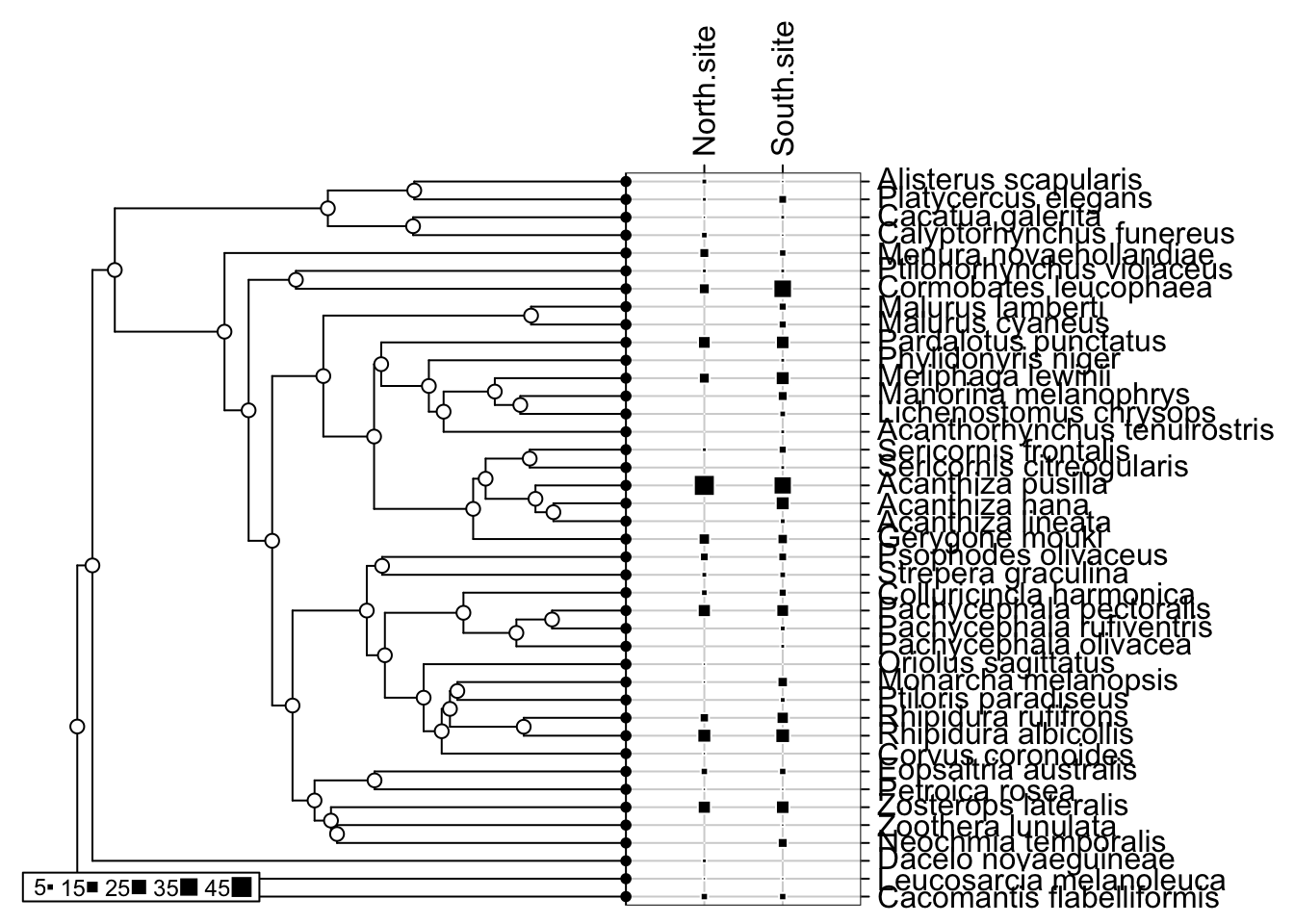For is data, the following commands display basic data information and run the `iNextPD()` function for `q = 0`.

``iNextPD(x=bird\$abun, labels=bird.lab, phy=bird.phy, q=0, datatype="abundance")``

The `iNextPD()` function returns the `"iNextPD"` object including three data frames: `\$DataInfo` for summarizing data information; `\$iNextPDEst` for showing phylogenetic diversity estimates along with related statistics for a series of rarefied and extrapolated samples; and `\$AsyPDEst` for showing asymptotic phylogenetic diversity estimates along with related statistics. `\$DataInfo`, as shown below, returns basic data information including the reference sample size (`n`), observed species richness (`S.obs`), a sample coverage estimate (`SC`), and the first ten frequency counts (`f1‐f10`). This part of output can also be computed by the function `iNEXT::DataInfo()`

``````\$DataInfo: basic data information
site   n S.obs     SC f1 f2 f3 f4 f5 f6 f7 f8 f9 f10
1 North.site 202    27 0.9705  6  4  2  2  2  0  1  1  1   0
2 South.site 307    38 0.9838  5  6  3  2  3  4  2  0  2   2``````

For incidence data, the list `\$DataInfo` includes the reference sample size (`T`), observed species richness (`S.obs`), total number of incidences (`U`), a sample coverage estimate (`SC`), and the first ten incidence frequency counts (`Q1‐Q10`).

In the `North.site`, by default, 40 equally spaced knots (samples sizes) between 1 and 404 (= 2 x 202, double reference sample size) are selected. Diversity estimates and related statistics are computed for these 40 `knots` (corresponding to sample sizes m = 1, 12, 23, … 202, …, 404), which locates the reference sample at the mid‐point of the selected knots. By default we only show five estimates on the screen, user should call `iNextPD.object\$iNextPDEst` to show complete output. If the argument `se=TRUE`, then the bootstrap method is applied to obtain the `conf` (by default `conf=0.95`) confidence intervals for each diversity and sample coverage estimates.

For the sample size corresponding to each knot, the list `\$iNextPDEst` (as shown below for the `North.site`) includes the sample size (`m`, i.e., each of the 40 knots), the method (`interpolated`, `observed`, or `extrapolated`, depending on whether the size `m` is less than, equal to, or greater than the reference sample size), the diversity order, the diversity estimate of order q (`qPD`), the 95% lower and upper confidence limits of diversity (`qD.95.LCL`, `qD.95.UCL`), and the sample coverage estimate (`SC`) along with the 95% lower and upper confidence limits of sample coverage (`SC.95.LCL`, `SC.95.UCL`). These sample coverage estimates with `conf`% confidence intervals are used for plotting the sample completeness curve and coverage-based R/E curves.

``````\$iNextPDEst: phylogenetic diversity estimates with rarefied and extrapolated samples.
\$North.site
m       method order      qPD qPD.95.LCL qPD.95.UCL    SC SC.95.LCL SC.95.UCL
1    1 interpolated     0   82.858     75.467     90.248 0.080     0.059     0.100
10 101 interpolated     0 1043.756    961.640   1125.873 0.935     0.915     0.954
20 202     observed     0 1222.098   1091.198   1352.998 0.970     0.952     0.989
30 298 extrapolated     0 1299.178   1125.847   1472.508 0.984     0.967     1.000
40 404 extrapolated     0 1337.896   1122.961   1552.832 0.992     0.978     1.000

NOTE1: Only show five estimates, call iNextPD.object\$iNextPDEst to show complete output.``````

`\$AsyPDEst` lists the observed diversity, asymptotic estimates, estimated bootstrap s.e. and 95% confidence intervals for Hill numbers with q = 0, 1, and 2. See Hsieh and Chao (2016) for asymptotic estimators. The output for the bird data is shown below. All row and column variables are self‐explanatory.

``````\$AsyPDEst: asymptotic phylogenetic diversity estimates along with related statistics.
Observed Estimator Est_s.e. 95% Lower 95% Upper

North.site q = 0  1222.098  1367.729  139.957  1222.098  1642.039
q = 1   437.248   456.014   23.520   437.248   502.113
q = 2   212.398   214.063   10.678   212.398   234.992
South.site q = 0  1416.719  1561.469   87.287  1416.719  1732.548
q = 1   455.438   469.981   20.677   455.438   510.508
q = 2   205.894   206.898    8.704   205.894   223.958``````

To show the completed branch abundance/incience and branch length (Ui, Li), i = 1, 2, …, B, user could call `iNextPD.object\$ExpandData`.

In practice, the user may specify an integer sample size for the argument `endpoint` to designate the maximum sample size of R/E calculation. For Faith’s PD, the extrapolation method is reliable up to the double reference sample size; beyond that, the prediction bias may be large. However, for measures of q = 1 and 2, the extrapolation can usually be safely extended to the asymptote if data are not sparse; thus there is no limit for the value of `endpoint` for these two measures.

The user may also specify the number of `knots` in the range of sample size between 1 and the endpoint. If you choose a large number of knots, then it may take a long time to obtain the output due to the time‐consuming bootstrap method. Alternatively, the user may specify a series of sample sizes for R/E computation, as in the following example:

``````# set a series of sample sizes (m) for R/E computation
m <- c(1, 5, 20, 50, 100, 200, 400)
iNextPD(x=bird\$abun, labels=bird.lab, phy=bird.phy,
q=0, datatype="abundance", size=m)``````

Further, `iNextPD` can simultaneously run R/E computation for Hill numbers with q = 0, 1, and 2 by specifying a vector for the argument `q` as follows:

``````out <- iNextPD(x=bird\$abun, labels=bird.lab, phy=bird.phy,
q=c(0,1,2), datatype="abundance", size=m)``````

## POINT ESTIMATION FUNCTION: estimatePD()

We also supply the function

``````estimatePD(x, labels, phy, datatype="abundance", base="size",
level=NULL, conf=0.95, digits=4) ``````

to compute diversity estimates with q = 0, 1, 2 for any particular level of sample size (`base="size"`) or any specified level of sample coverage (`base="coverage"`) for either abundance data (`datatype="abundance"`) or incidence data (`"incidence_raw"`). If `level=NULL`, this function computes the diversity estimates for the minimum sample size/coverage among all sites.

For example, the following command returns the species diversity with a specified level of sample coverage of 97.5% for the bird abundance-based data. For some sites, this coverage value corresponds to the rarefaction part whereas the others correspond to extrapolation, as indicated in the method of the output.

``````estimatePD(bird\$abun, bird.lab, bird.phy, "abundance",
base="coverage", level=0.975, conf=0.95)
site        m       method order    SC       qPD qPD.95.LCL qPD.95.UCL
1  North.site 227.0711 extrapolated     0 0.975 1248.1118  1128.6005  1367.6232
3  North.site 227.0711 extrapolated     1 0.975  439.4657   386.8856   492.0458
5  North.site 227.0711 extrapolated     2 0.975  212.5806   179.6321   245.5291
8  South.site 247.8890 interpolated     0 0.975 1367.1348  1288.1097  1446.1600
10 South.site 247.8890 interpolated     1 0.975  451.9783   412.1321   491.8246
12 South.site 247.8890 interpolated     2 0.975  205.6565   176.3483   234.9647``````

## GRAPHIC DISPLAYS: FUNCTION `ggiNEXT()`

The function `ggiNEXT()`, which extends `ggplot2` to the `"iNextPD"` object with default arguments, is described as follows:

``ggiNEXT(x, type=1, se=TRUE, facet.var="none", color.var="site", grey=FALSE)  ``

Here `x` is an `"iNextPD"` object. Three types of curves are allowed:

1. Sample-size-based R/E curve (`type=1`): this curve plots diversity estimates with confidence intervals (if `se=TRUE`) as a function of sample size up to double the reference sample size, by default, or a user‐specified `endpoint`.

2. Sample completeness curve (`type=2`) with confidence intervals (if `se=TRUE`): this curve plots the sample coverage with respect to sample size for the same range described in (1).

3. Coverage-based R/E curve (`type=3`): this curve plots the diversity estimates with confidence intervals (if `se=TRUE`) as a function of sample coverage up to the maximum coverage obtained from the maximum size described in (1).

The argument `facet.var=("none", "order", "site" or "both")` is used to create a separate plot for each value of the specified variable. For example, the following code displays a separate plot for each value of the diversity order q. The user may also use the argument `grey=TRUE` to plot black/white figures. The usage of color.var is illustrated in the incidence data example described in later text. The `ggiNEXT()` function is a wrapper around `ggplot2` package to create a R/E curve using a single line of code. The resulting object is of class `"ggplot"`, so can be manipulated using the `ggplot2` tools.

``````out <- iNextPD(bird\$abun, bird.lab, bird.phy,
q=c(0, 1, 2), datatype="abundance", endpoint=400)

# Sample‐size‐based R/E curves, separating by "site""
ggiNEXT(out, type=1, facet.var="site")
## Not run:
# Sample‐size‐based R/E curves, separating by "order"
ggiNEXT(out, type=1, facet.var="order")
# display black‐white theme
ggiNEXT(out, type=1, facet.var="order", grey=TRUE)
## End(Not run)``````

The argument `facet.var="site"` in `ggiNEXT` function creates a separate plot for each site as shown below:

``````# Sample‐size‐based R/E curves, separating by "site""
ggiNEXT(out, type=1, facet.var="site")``````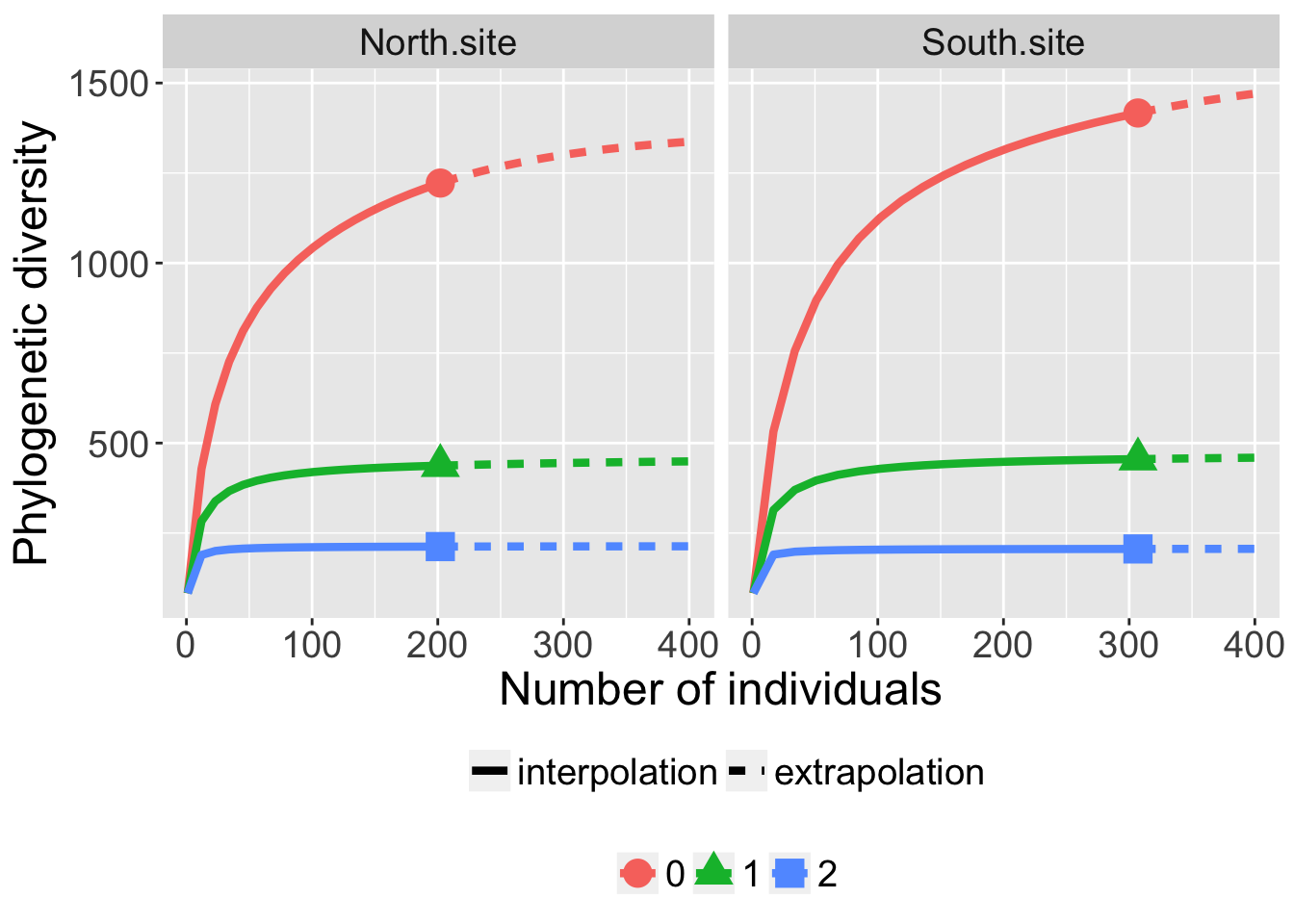The argument `facet.var="order"` and `color.var="site"` creates a separate plot for each diversity order site, and within each plot, different colors are used for two sites.

``ggiNEXT(out, type=1, facet.var="order", color.var="site")``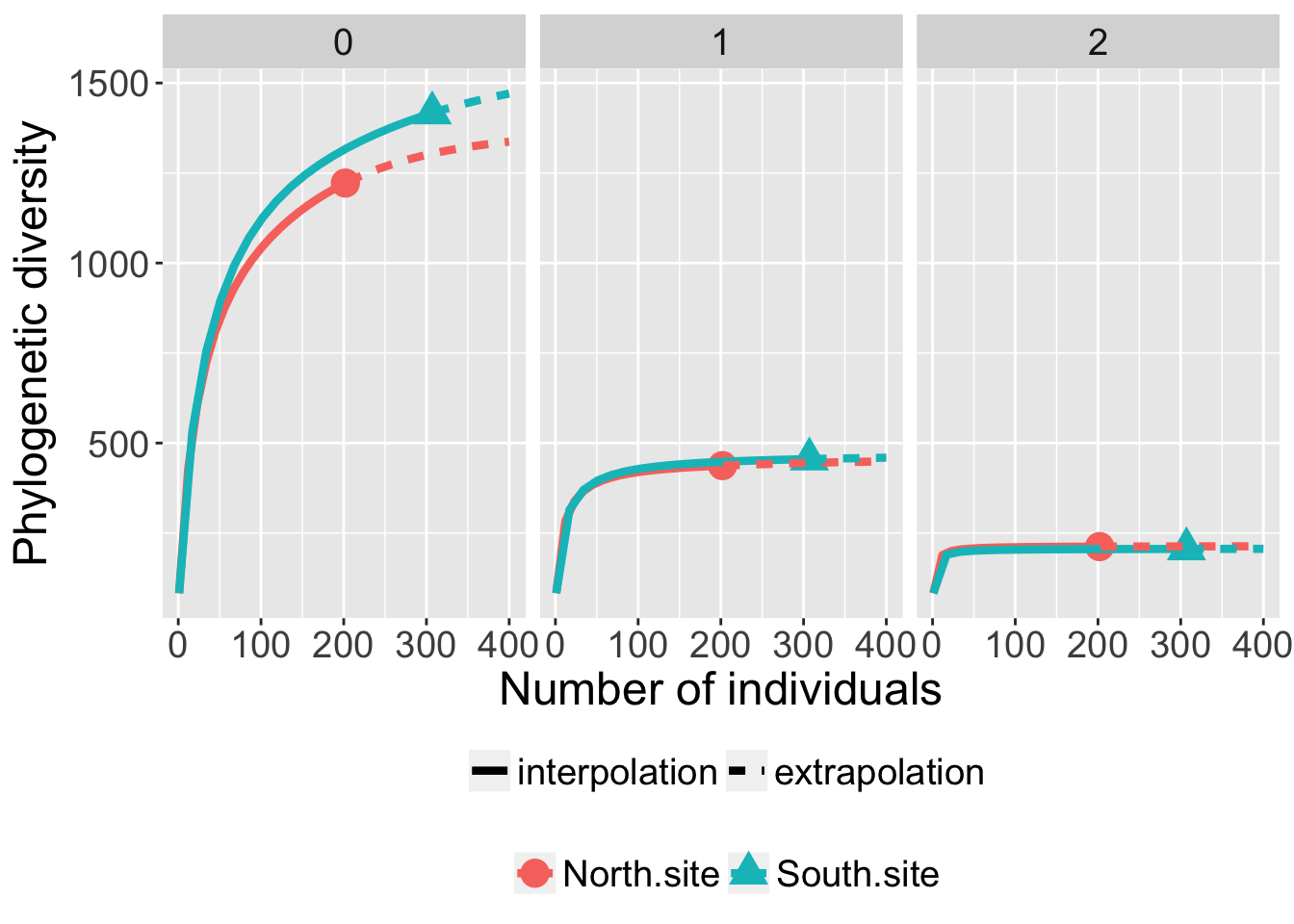The following commands return the sample completeness curve in which different colors are used for the two sites:

``ggiNEXT(out, type=2, facet.var="none", color.var="site")``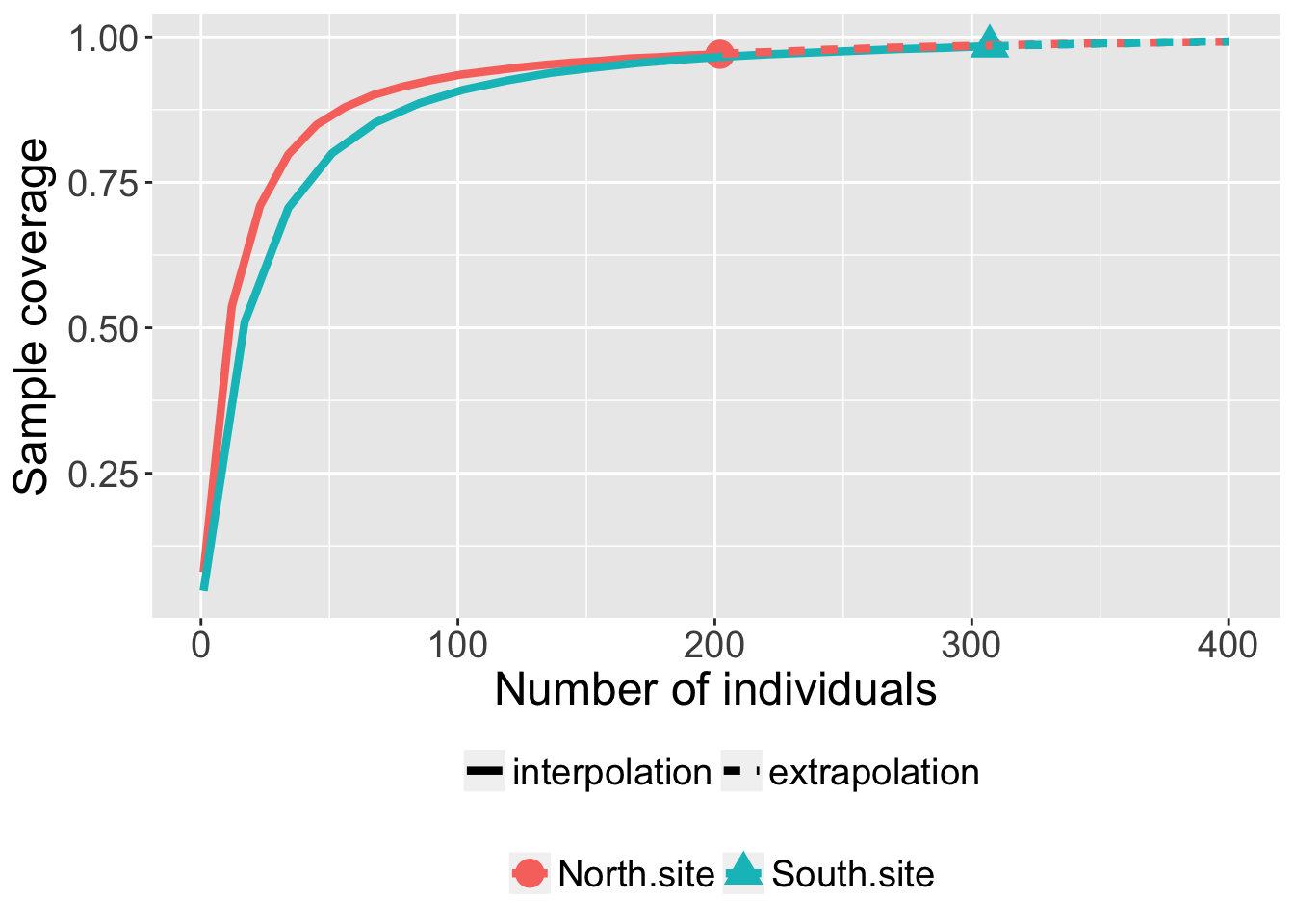The following commands return the coverage‐based R/E sampling curves in which different colors are used for the two sites (`facet.var="site"`) and for three orders (`facet.var="order"`)

``ggiNEXT(out, type=3, facet.var="site")``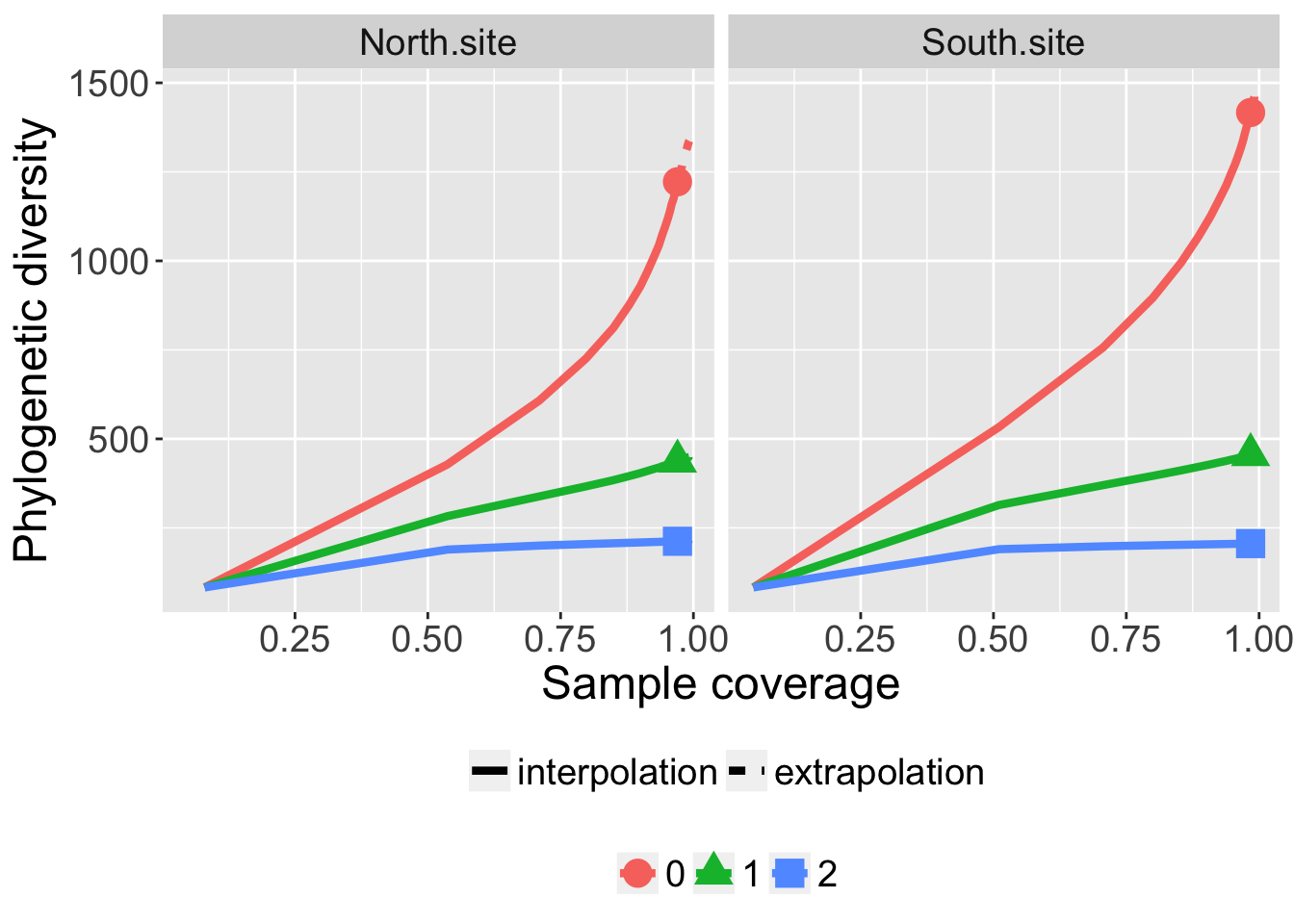``ggiNEXT(out, type=3, facet.var="order", color.var="site")``